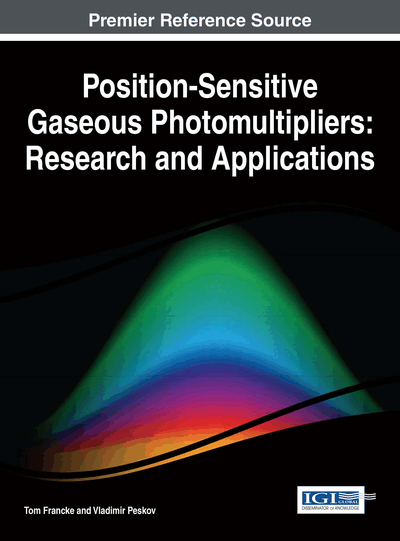# Electron Multiplication and Electron Multipliers

DOI: 10.4018/978-1-5225-0242-5.ch002

## Abstract

This chapter describes the process of electron avalanche multiplication, which is the only method known to detect the smallest possible electrical signal, a single electron at rest. Electron avalanche multiplication can advantageously be used in position sensitive photomultipliers to with high efficiency detect single photons with respect to position, time, direction and energy.
Chapter Preview
Top

## 1. Electron Avalanche Multiplication In Gases

Gaseous photomultipliers can detect singe photoelectrons due to avalanche multiplication in a gas where a large number of secondary electrons are created from a single photoelectron. Avalanche multiplication is an interesting phenomenon discovered a long time ago by Townsend (Townsend, 1901; Townsend, 1903a; Townsend, 1903b). In 1908 Rutherford and Geiger for the first time exploited electron avalanche multiplication in a practical device to detect alpha particles (Rutherford, 1908).

Let us first consider electron avalanche development in a uniform electric field. In this field a free electron is liberated, created by external radiation e.g. by photoelectric effect between a single photon and a gas which the electric field permeates in. If the electric field is strong enough, the single primary electron can gain sufficient kinetic energy Ek from the electric field to experience inelastic collisions with atoms or molecules in the gas.

If Ek > Ei, where Ei is the ionization potential of the gas atoms or molecules, a secondary electron can be created ionization of the gas in these collisions. This secondary electron, as well as the initial electron, gain further kinetic energy in the electric field, and after drifting some distance dx in the gas and again gaining a kinetic energy Ek > Ei, they collide with the gas atoms and produce further secondary electrons through ionization. An electron avalanche is triggered. This process can be described by the differential equation:dne = α ne(x) dx, (1) where ne is the number of secondary electrons and α is a coefficient often called a first Townsend coefficient.

The number of secondary electrons grows exponentially with the distance x:

ne= exp(αx), (2).

The valueA = exp(αx), (3) represents the multiplication factor or the gas gain. As can be seen the avalanche just increases and increases as long as it can propagate.

The Townsend coefficient, α, depends on the electric field strength, E, and the gas pressure, p. There are several parametric descriptions of the first Townsend coefficient (Aoyama, 1985). Most of them satisfactorily describe the experimental data in some ranges of E/p, with correctly determined parameters. The generalized form of the reduced first Townsend coefficient, α/p, is given by the expression:α/p = A exp(−B p/E), (4),where A and B are parameters depending on the gas and the E/p range.

In the avalanche cloud, electrons drift two-tree orders of magnitude faster than the positive ions due to the much higher mass and larger interaction cross section of the ions (the electric field strength is the same for electrons and ions).So, during the avalanche development ions can be considered to a good approximation to be stationary. This is illustrated in Figure 1. First, an exponentially growing number of electrons are created and move fast towards the positive anode. They induce a fast and short pulse in the readout electrodes. When the electron pulse is gone, a cloud of positive ions slowly move towards the cathode producing a long and slow pulse.

Figure 1.

The development of a Townsend avalanche; the electrons move 100-1000 times faster than the heavier ions so the avalanche process is separated into two distinctive events separated in time. First, an exponentially growing number of electrons are created and move fast towards the positive anode. They induce a fast and short pulse in the readout electrodes. When the electron pulse is gone, a cloud of positive ions slowly move towards the cathode producing a long and slow pulse.

## Complete Chapter List

Search this Book:
Reset